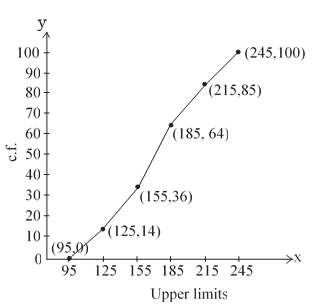#### The following is the frequency distribution of duration for100 calls made on a mobile phone : Duration (in seconds) Number of calls 95-125 125-155 155-185 185-215 215-245 14 22 28 21 15 Calculate the average duration (in sec) of a call and also find the median from cumulative frequency curve.Solution.

 Duration fi xi fiui 95-125 14 110 -2 -28 125-155 22 140 -1 -22 155=185 28 170 = a 0 0 185-215 21 200 1 21 215-245 21 230 2 30

a = 170, h = 30
Average =

 less than type Duration Number of calls less than 95 less than 125 less than 155 less than 185 less than 215 less than 245 0 0+14 = 14 14+22 =36 36 + 28 = 64 64 + 21 = 85 85 + 15 =100n = 100

median is 170